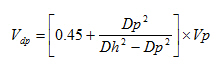## Determine surge and swab pressure method 2 Calculation Example

This section will show to how to use the formula from the previous topic, surge and swab pressure calculation 2nd method , to determine surge and swab pressure. I still use the same parameters as I used for the first method of surge and swab pressure calculation so you can compare the result between method#1 and method#2.

The well information is listed below:

θ300 = 85

θ600 = 130

Hole diameter = 9 inch

Drill Collar = 6.25 inch

ID of drill collar = 2.5 inch

Drillpipe diameter = 5 inch

ID of drill pipe = 3.5 inch

Average pipe running or pulling speed = 250 ft/min

Drill Pipe Length = 12000 ft

Drill Collar Length = 800 ft

Current Mud Weight = 12.5 ppg

Well TVD = 9000 ft

## Determine surge and swab pressure method 2

This is another method for calculating surge and swab pressure. With this method, there are 3 assumptions as follows:

• Closed ended pipe (plug flow)

• Laminar flow around drill pipe

• Turbulent flow around drill collar

How to determine surge and swab pressure with this method

1. Determine pressure around drill pipe

2. Determine pressure around drill collar

Note: The calculation methodology is different from the first method because both pressure losses are determined by separate set of equations.

3. Determine total pressure loss by summation of step 1 and step 2

4. Determine surge and swab pressure

### Determine pressure loss around drill pipe by following calculations

1. Determine fluid velocity around drill pipeWhere;

Vdp is the fluid velocity around drill pipe in ft/min.

Vp is pipe movement velocity in ft/min.

Dp is drill pipe diameter in inch.

Dh is hole diameter in inch.

## Determine surge and swab pressure for close-ended pipe

I would like to show more calculation about surge and swab pressure. In this article, I will show how to use the previous formulas (Surge and swab pressure method 1) to figure out surge and swab pressure for close-ended pipe (plug flow). The calculation concept is pretty much the same but I would like to show you how much difference between surge/swab if you have the close-ended pipe.

I still use the same data but this time so I can compare apple to apple with the previous calculation.

Example

The well information is listed below:

θ300 = 85

θ600 = 130

Hole diameter = 9 inch

Drill Collar = 6.25 inch

ID of drill collar = 2.5 inch

Drillpipe diameter = 5 inch

ID of drill pipe = 3.5 inch

Average pipe running or pulling speed = 250 ft/min

Drill Pipe Length = 12000 ft

Drill Collar Length = 800 ft

Current Mud Weight = 12.5 ppg

Well TVD = 9000 ft

## Determine surge and swab pressure for open-ended pipe

You’ve learn how to determine surge and swab pressure from the previous topic (Surge and swab pressure method 1) and this topic will demonstrate you how to use those equations to determine surge and swab pressure for the open-ended pipe.

Example

The well information is listed below:

θ300 = 85

θ600 = 130

Hole diameter = 9 inch

Drill Collar = 6.25 inch

ID of drill collar = 2.5 inch

Drillpipe diameter = 5 inch

ID of drill pipe = 3.5 inch

Average pipe running or pulling speed = 250 ft/min

Drill Pipe Length = 12000 ft

Drill Collar Length = 800 ft

Current Mud Weight = 12.5 ppg

Well TVD = 9000 ft

## Surge and Swab Calculation Method 1

This is the first method to determine surge and swab pressure.

Surge is additional pressure due to pipe movement downward and swab is reduction of pressure due to upward movement of drill string.Bottom hole pressure is reduced due to swabbing effect.Bottom hole pressure is increased due to surging effect.